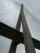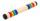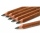# Wood dividing

Which equation calculates the number of 1/3-foot pieces that can be cut from a piece of wood that is 7 feet long?

Result

n =  21

#### Solution:

n*(1/3)=7

n = 21

n = 21

Calculated by our simple equation calculator.

Leave us a comment of example and its solution (i.e. if it is still somewhat unclear...):

Showing 0 comments:Be the first to comment!#### To solve this example are needed these knowledge from mathematics:

Do you have a linear equation or system of equations and looking for its solution? Or do you have quadratic equation? Do you want to convert length units?

## Next similar examples:

1. Number with onesThe first digit of the number is 1, if we move this digit to the end we get a 3 times higher number, which is the number?
2. Unknown number 11That number increased by three equals three times itself?
3. Dropped sheetsThree consecutive sheets dropped from the book. The sum of the numbers on the pages of the dropped sheets is 273. What number has the last page of the dropped sheets?
4. Unknown numberI think the number. I'll reduce it to its one-third. The result is then increased by one-third, and I get the number 12.
5. Bridge piersOne quarter of the bridge pier is sunk into the ground. Two thirds are in the water. Protruding above the water is 1.20 m long. Determine the height of bridge piers.
6. NormThree workers planted 3555 seedlings of tomatoes in one dey. First worked at the standard norm, the second planted 120 seedlings more and the third 135 seedlings more than the first worker. How many seedlings were standard norm?
7. Simple equationSolve the following simple equation: 2. (4x + 3) = 2-5. (1-x)
8. Equation 29Solve next equation: 2 ( 2x + 3 ) = 8 ( 1 - x) -5 ( x -2 )
9. Simple equationSolve for x: 3(x + 2) = x - 18
10. Find xSolve: if 2(x-1)=14, then x= (solve an equation with one unknown)
11. Negative in equation2x + 3 + 7x = – 24, what is the value of x?
12. Forest nurseryIn the forest nursery after winter, they found that 1/10 stems died out of them. For them, they land 193 new spruces. How many spruces are in the forest nursery?
13. BarHalf of the bar is green, the third is yellow and the remaining 40cm is red. How long is a bar?
14. Customary lengthConvert length 65yd 2 ft to ft
15. Pencil cutVeronika cut 10cm pencil. Each turn, the pencil was reduced by 0.2mm. She turned the pencil 100 times. How many centimeters has a pencil now?
16. StepsWalkway from the house to the school is 180 m long. How many more steps Michael makes than his father on the way to school, if the length of Michael's step is 60 cm and the length of his father's step is 90 cm.
17. RecipeA recipe requires 2 pounds of flour. If a chef wants to triple the recipe, how many ounces of flour will be needed?